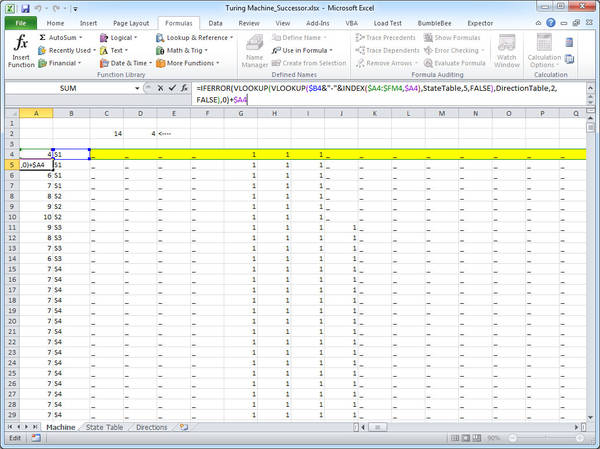# Implementing a Turing machine in ExcelFelienne describes how she, Daan van Berkel and some other friends went away for a weekend to hack a Turing machine out of Excel formulas. Lacking an infinitely long tape, they had to kludge around a bit, but the outcome is both cool and instructional (here's the machine itself). The Turing Machine is Alan Turing's "hypothetical device that manipulates symbols on a strip of tape," which formed the basis for modern, general-purpose computers.

Then, lets have a look at the machine itself. Since we are only using formulas, we cannot continuously change the tape. Therefore, we use one row in the spreadsheet to represent one state of the tape. Each following line represents the state of the tape after one transition. As Willem van de Ende poetically put it: "No state was harmed in the making of this Turing Machine"

This means the top most line of the spreadsheet (shown in yellow in the following screen shot) represents this initial state of the machine. C2 shows the row where we reach the halting state and D2 counts the number of 1′s from there. As the first line contains three 1′s, this machine indeed seems to be calculating the successor.

Now, how do we get from one state to the other? For this we need to do some administration. In column A, we save the column position of the head. In column B, we save the current state. With that we can calculate the value of one square on the tape, by looking at the state and the previous value on the tape, with the formula below:

(via Hacker News)